feedback

Feedback connection of multiple models

Description

example

sys = feedback(sys1,sys2) returns a model object sys for the negative feedback interconnection of model objects sys1,sys2.From the figure, the closed-loop model sys has u as input vector and y as output vector. Both models, sys1 and sys2, must either be continuous or discrete with identical sample times.

example

sys = feedback(sys1,sys2,feedin,feedout) computes a closed-loop model sys using the input and output connections specified using feedin and feedout. Use this syntax when you want to connect only a subset of the available I/Os of MIMO systems.

example

sys = feedback(sys1,sys2,'name') computes a closed-loop model sys with the feedback connections specified by the respective I/O names of MIMO models sys1 and sys2. Use the 'name' flag only when all the required I/Os in the set of MIMO systems are properly named.

example

sys = feedback(___,sign) returns a model object sys for a feedback loop with the type of feedback specified by sign. By default, feedback assumes negative feedback and is equivalent to feedback(sys1,sys2,-1). To compute the closed-loop system with positive feedback, use sign = +1.

Examples

collapse all

pendulumModelAndController.mat contains a SISO inverted pendulum transfer function model G and its associated PID controller C.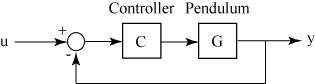Load the inverted pendulum and controller model to the workspace.

size(G)
Transfer function with 1 outputs and 1 inputs.
size(C)
PID controller with 1 output and 1 input.

Use feedback to create the negative feedback loop with G and C.

sys = feedback(G*C,1)
sys =

1.307e-06 s^3 + 3.136e-05 s^2 + 5.227e-06 s
---------------------------------------------------------
2.3e-06 s^4 + 1.725e-06 s^3 - 4.035e-05 s^2 - 5.018e-06 s

Continuous-time transfer function.

sys is the resultant closed loop continuous-time transfer function obtained using negative feedback. feedback converts the PID controller model C to a transfer function before connecting it to the continuous-time transfer function model G. For more information, see Rules That Determine Model Type.

For this example, consider two transfer functions that describe a plant G and controller C respectively.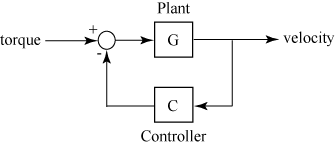$G\left(s\right)=\frac{2{s}^{2}+5s+1}{{s}^{2}+2s+3}\phantom{\rule{0.2777777777777778em}{0ex}}\phantom{\rule{0.2777777777777778em}{0ex}}\phantom{\rule{0.2777777777777778em}{0ex}}\phantom{\rule{0.2777777777777778em}{0ex}}\phantom{\rule{0.2777777777777778em}{0ex}}\phantom{\rule{0.2777777777777778em}{0ex}}C\left(s\right)=\frac{5\left(s+2\right)}{s+10}$

Create the plant and controller transfer functions.

G = tf([2 5 1],[1 2 3],'inputname',"torque",'outputname',"velocity");
C = tf([5,10],[1,10]);

Use feedback to create the negative feedback loop using G and C.

sys = feedback(G,C,-1)
sys =

From input "torque" to output "velocity":
2 s^3 + 25 s^2 + 51 s + 10
---------------------------
11 s^3 + 57 s^2 + 78 s + 40

Continuous-time transfer function.

sys is the resultant closed loop transfer function obtained using negative feedback with torque as the input and velocity as the output.

For this example, consider two transfer functions that describe a plant G and controller C respectively.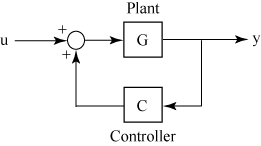$G\left(s\right)=\frac{2{s}^{2}+5s+1}{{s}^{2}+2s+3}\phantom{\rule{0.2777777777777778em}{0ex}}\phantom{\rule{0.2777777777777778em}{0ex}}\phantom{\rule{0.2777777777777778em}{0ex}}\phantom{\rule{0.2777777777777778em}{0ex}}\phantom{\rule{0.2777777777777778em}{0ex}}\phantom{\rule{0.2777777777777778em}{0ex}}C\left(s\right)=\frac{5\left(s+2\right)}{s+10}$

Create the plant and controller transfer functions.

G = tf([2 5 1],[1 2 3],'inputname',"torque",'outputname',"velocity");
C = tf([5,10],[1,10]);

Use feedback to create the positive feedback loop using G and C.

sys = feedback(G,C,+1)
sys =

From input "torque" to output "velocity":
-2 s^3 - 25 s^2 - 51 s - 10
---------------------------
9 s^3 + 33 s^2 + 32 s - 20

Continuous-time transfer function.

sys is the resultant closed loop transfer function obtained using positive feedback with torque as the input and velocity as the output.

Based on the figure below, consider connecting two MIMO transfer functions with two inputs and two outputs in a negative feedback loop.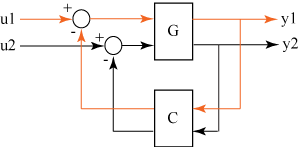For this example, create two random continuous state-space models using rss.

size(G)
State-space model with 2 outputs, 2 inputs, and 4 states.
size(C)
State-space model with 2 outputs, 2 inputs, and 2 states.

Use feedback to connect the two state-space models in a negative feedback loop according to the above figure.

sys = feedback(G,C,-1);
size(sys)
State-space model with 2 outputs, 2 inputs, and 6 states.

The resulting state-space model sys is a 2 input, 2 output model with 6 states. The negative feedback loop is completed such that,

• The first output of G is connected to the first input of C

• The second output of G is connected to the second input of C

mimoPlantAndController.mat contains a 2 input, 2 output transfer function plant model G and a 2 input, 2 output transfer function controller model C to be connected as follows: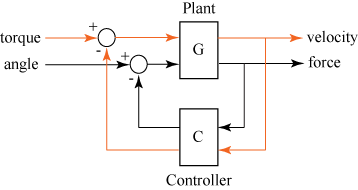First, load the plant and controller models to the workspace.

size(G)
Transfer function with 2 outputs and 2 inputs.
size(C)
Transfer function with 2 outputs and 2 inputs.

By default, feedback would connect the first output of G to the first input of C and the second output of G to the second input of C. In order to connect the plant and controller according to the figure, name the respective I/Os of the two systems to ensure the correct connections.

G.InputName
ans = 2x1 cell
{'torque'}
{'angle' }

G.OutputName
ans = 2x1 cell
{'velocity'}
{'force'   }

C.InputName
ans = 2x1 cell
{'force'   }
{'velocity'}

C.OutputName
ans = 2x1 cell
{'angle' }
{'torque'}

Then use the 'name' flag with the feedback command to make the connections according to the I/O names.

sys = feedback(G,C,'name');

The resulting closed loop negative feedback transfer function sys has the feedback connections in the required order.

Consider a state-space plant G with five inputs and four outputs and a state-space feedback controller K with three inputs and two outputs. The outputs 1, 3, and 4 of the plant G must be connected the controller K inputs, and the controller outputs to inputs 2 and 4 of the plant.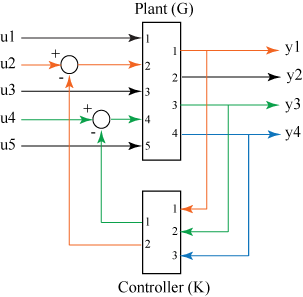For this example, generate randomized continuous-time state-space models using rss for both G and K.

Define the feedout and feedin vectors based on the inputs and outputs to be connected in a feedback loop.

feedin = [2 4];
feedout = [1 3 4];
sys = feedback(G,K,feedin,feedout,-1);
size(sys)
State-space model with 4 outputs, 5 inputs, and 6 states.

sys is the resultant closed loop state-space model obtained by connecting the specified inputs and outputs of G and K.

Input Arguments

collapse all

Systems to connect in a feedback loop, specified as dynamic system models. Dynamic systems that you can use include:

• Continuous-time or discrete-time numeric LTI models such as tf, zpk, pid, pidstd, or ss models.

• Frequency response models such as frd or genfrd.

• Generalized or uncertain LTI models such as genss or uss (Robust Control Toolbox) models. (Using uncertain models requires Robust Control Toolbox™ software.)

The resulting feedback loop assumes

• current values of the tunable components for tunable control design blocks.

• nominal model values for uncertain control design blocks.

When sys1 and sys2 are two different model types, feedback uses precedence rules to determine the resulting model sys. For example, when a state-space model and a transfer function is connected in a feedback loop, the resulting system is a state-space model based on the precedence rules. For more information, see Rules That Determine Model Type.

Subset of inputs to be used, specified as a vector.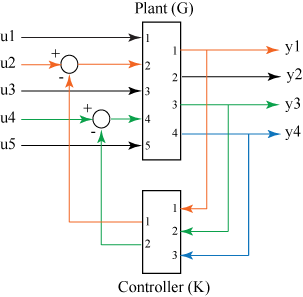From the figure, feedin contains indices of the input vector of MIMO plant P and specifies which subset of inputs u are involved in the feedback loop. The resulting model sys has the same inputs as G, with their orders preserved.

For an example, see Specify Input and Output Connections in a Feedback Loop.

Subset of outputs to be used, specified as a vector.

feedout specifies which outputs of MIMO plant G are used for feedback. The resulting model sys has the same outputs as G, with their orders preserved.

For an example, see Specify Input and Output Connections in a Feedback Loop.

Type of feedback, specified as -1 for negative feedback or +1 for positive feedback. feedback assumes negative feedback by default.

Output Arguments

collapse all

Closed-loop system, returned as a SISO, or MIMO dynamic system model. sys can be one of the following depending on the precedence rules:

• Continuous-time or discrete-time numeric LTI models, such as tf, zpk, ss, pid, or pidstd models.

• Generalized or uncertain LTI models such as genss or uss (Robust Control Toolbox) models. (Using uncertain models requires Robust Control Toolbox software.)

When sys1 and sys2 are two different model types, feedback uses precedence rules to determine the resulting model sys. For example, when a state-space model and a transfer function is connected in a feedback loop, the resulting system is a state-space model based on the precedence rules outlined in Rules That Determine Model Type.

Limitations

• The feedback connection must be free of algebraic loops. For instance, if D1 and D2 are the feedthrough matrices of sys1 and sys2, this condition is equivalent to:

• I + D1D2 nonsingular when using negative feedback

• I − D1D2 nonsingular when using positive feedback